## Monday, February 27, 2012

### Coefficients of Contraction/Expansion at Bridges.

Written by Chris Goodell, P.E., D. WRE | WEST Consultants

In HEC-RAS, it is a well known modeling technique to increase the coefficients of contraction and expansion in the vicinity of a bridge for steady flow modeling.
This is done to capture the energy loss resulting from increased flow contraction approaching the bridge, and increased flow expansion when leaving the bridge.  This energy loss is not accounted for in the friction loss, so HEC has added in the ability to account for it using the contraction and expansion coefficients, multiplied by the difference in velocity head between two cross sections.  Typically, RAS modelers will apply the higher coefficients (0.3 for contraction, 0.5 for expansion) at Cross Sections 4, 3, and 2 of the traditional cross section layout for bridges (see figure at the bottom of this post).  Cross Section number 1 (the most downstream of the 4-cross section layout) is typically left at the default values of 0.1 and 0.3, respectively.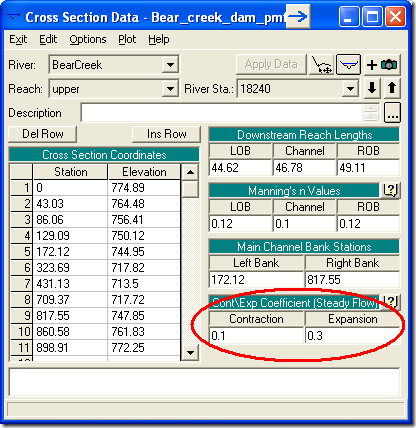A common question is “why is Cross Section #1 left with the default values?”
The coefficients of contraction and expansion are applied to the reach from the cross section at which they are defined to the next cross section downstream.  In the energy equation,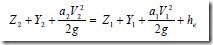, he represents the head loss from one cross section to the next.  The equation for head loss, he, is: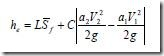Where C is the coefficient of contraction or expansion.  Subscripts 1 and 2 represent the two neighboring cross sections.  So here you can see clearly that the coefficient of contraction or expansion is applied over a reach, defined by L, which is the length between Cross Sections 1 and 2.
So, for bridge modeling, the reach from Cross Section 4 to 3 defines the zone of contraction as flow approaches the bridge.  The higher coefficients are applied to Cross Section 4 in this case.
The reach from Cross Section 3 to 2 defines the fully contracted zone though the bridge.  You could make a case that since the flow is fully contracted in this zone, that the typical coefficients should be used (0.1 and 0.3).  However, since there is usually a higher amount of turbulence in this zone, traditionally everyone keeps the higher coefficients (0.3 and 0.5).  The higher coefficients are applied to Cross Section 3 in this case.
The reach from Cross Section 2 to 1 defines the expansion zone downstream of the bride.  The higher coefficients are applied to cross section 2 in this case.
At Cross Section 1 and further on downstream, the flow is considered fully expanded, so Cross Section 1 maintains the typical coefficients (0.1 and 0.3).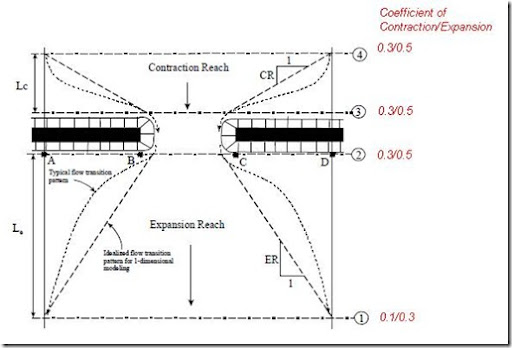1.I have a bridge, and there is a new bridge desigfned some 35 m from the actual. The piles of the new bridge are not aligned with the existing ones. I´m doing a sensibility of the contraction/expansion coeficients. Is there any publication of suggested values for coeficients in this case? One question besides the modeling. Is there a criteria for the piers protection in the case? What is the protection of the upstream pile overlap part of the protection of the downstreampile? the CAD file show the distribution of the piles and the bridges

2.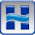In your case, I would probably use the higher values suggested for bridges in the HEC-RAS manual. Not sure of any publications specifically on this matter.

3.I have assigned contraction & expansion coefficients of 0.3 and 0.5, respectively, at sections 2, 3, and 4; however, check-ras continues to give me the following error code:

"Contraction and expansion loss coefficients are 0.3 and 0.5, respectively. However, this cross section is not at a hydraulic structure."

Anyone else have this issue?

4.Never have, but it doesn't suprise me. If I recall, FEMA will accept a reasonable justification for ignoring a CheckRAS error code. Sounds like this is a good candidate.

5.How about culvert crossing? Will it be considered abrupt change with culvert crossing in comparison to bridge crossing? Will a higher loss coefficients 0.6 and 0.8 be applied to culvert crossing?

6.Yes. In fact the RAS manual suggests those higher contraction and expansion losses at culverts vs bridges.

7.Does Hec-Ras apply default contraction and expansion losses at internal bridge cross sections? I ask because I'm modeling a bridge that completely spans a 100-yr floodplain (No contraction expansion), yet I still get some inconsistencies between XC 3-upstream inside and downstream inside-XC 2.

1.The defaults everywhere in HEC-RAS is 0.1 and 0.3. If you have a bridge, generally you'll increase the coefficients at cross sections 2, 3, and 4 (and all intermediate cross sections in between) to 0.3 and 0.5. If you have a culvert with a significant constriction, go to 0.5 and 0.7. The inconsistencies you are seeing may be a result of ineffective flow trigger placement (both laterally and in elevation)and/or bank station placement. There may be some small differences, but the flow distribution inside the structure upstream and downstream should be similar.

2.Hi Chris
i am trying to run a pre vs post development model to assess the post dev impact.
The post dev model considers an earthworks platform for a warehouse( an obstruction to flow).
will it be justified to add contraction and expansions coefficients to the cross sections, similarly mentioned in the above comments?

Note i have tried (1)altering the terrain to create this platform obstruction and (2) inserted a conveyance obstruction, however both runs (1 and 2) show insignificant impacts to the stream flow. very different to what i expected to notice.

your feedback will be highly appreciated.

3.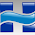Yes, I think it is justified.

8.Would it be necessary to adjust these coefficients for greenway bridges low in the floodplain that are typically flooded in a 100-year storm event? I've not seen anything done different and thought the default coefficients would be acceptable for a bridge located bank-to-bank that is over topped or completely submerged during the 100-year storm.

1.I think that's a logical approach.

9.Can hecras model handle an open channel and pipe in the same cross section. We have a storm pipe running parallel with river and was used to divert the certain amount of flow

10.Unfortunately no it cannot. But you could run a parallel reach with "lids" to handle that scenario. But keep in mind that RAS is not built for lins distance pressure flow.

11.Would it be recommended to leave the default contr/exp coefficients (0.1/0.3) for a flyover bridge with no abutments in the floodplain, but only piers? Thanks!

1.That would probably be okay as long as the piers are not too big relative to the bridge opening. However, why not bump them up to the standard for bridges-it will be more conservative? You can always try both (actually you should) to see how sensitive the results are to the contraction and expansion coefficients.

12.I have a floodplain model between two bridges. My cross sections 1,2,3, and 4 for bridge 1 justify using the recommended 0.3 and 0.5 expansion and contraction coefficients. However, for Bridge 2 we it is our assumption that section 4 is too far upstream to justify using a 0.3 contraction coefficient as the ratio between the effective flow and the total floodplain width is 0.4. We are leaning towards using the default contraction coefficient of 0.1 in this case. Any thoughts?

1.Sounds like a well-reasoned thought-out justification for using 0.1. Hard to argue with that.

13.I need to insert a HEC 2 dat file into HEC RAS.
The reach that I am reviewing is only a small portion of the HEC 2study.
Can I eliminate all the additoal reches and create the same output in HEC RAS? or - DO I NEED TO use THE ENTIRE REACH INSERTED IN THE
HEC RAS Model?
It is a existing FEMA Study that I need to assess encroachment by a proposed home near the FLOODWAY.

1.In theory yes you can do that. Just make sure to get the WS el from the cross section you wish to use as your new downstream boundary and use that for your boundary condition. Same thing with flow on the upstream end.

14.Regarding changing the coefficients at sections 2, 3, and 4. The HECRAS computations start downstream and progress upstream in a Steady Model. So it would seem the changes should occur a 1, 2, and 3.

1.You are right about the direction of computations, but the contraction and expansion coefficients are used to determine the form losses from the cross section they are defined at to the next cross section downstream. So, for example, while the computations might progress in the direction from cross section 3 to cross section 4, the losses in this reach are computed using the C&E coefficients, the Manning's n values, and the reach lengths defined at section 4.

15.I have a bridge that is well above the 100-year WSE and EGL but my model is showing a significant rise in cross-sections 3 and 4. Is there any way to get the model to show no rise?

16.I have a question about the evaluation of expansion reach lengths. I am going through the HEC-RAS 5.0.4 Application Guide, and reading about the procedure for checking and adjusting the expansion reach length. The guide provides equation 2-1 (page 2-23) for estimating the expansion reach length, based on the Froude number at cross-section 1 (fully expanded flow), the Froude number at cross-section 2 (just downstream of the bridge), the average length of obstruction by the bridge, and flow; (the respective values given are 0.30, 0.37, 470 ft, and 14000 cfs). I have plugged this into excel and can't match the example for the life of me. I get 765 ft, while the example calculates 778 feet. This is my equation in excel: Le=-298+257*(Fr2/Fr1)+0.918*(Lobs)+0.00479*(Q), where Le is the expansion length, Fr2 is the Froude number at cross-section 2 (0.37), Fr1 is the Froude number at cross-section 1 (0.30), Lobs is the average length of obstruction caused by the bridge (740 ft), and Q is the flow rate (14,000 cfs). Most likely I'm making an error somewhere, but wanted to see if anybody else gets similar results. I'd be really surprised if the Application Guide is incorrect.